Successfully reported this slideshow.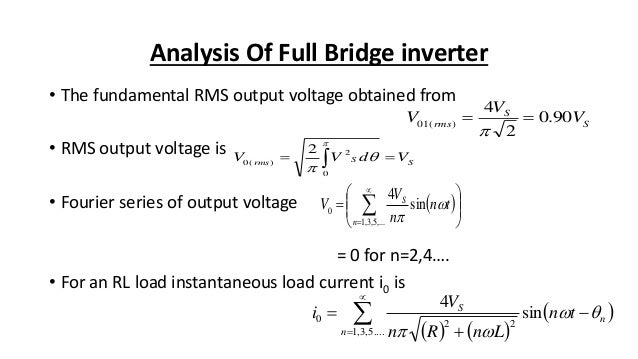Upcoming SlideShare
×

of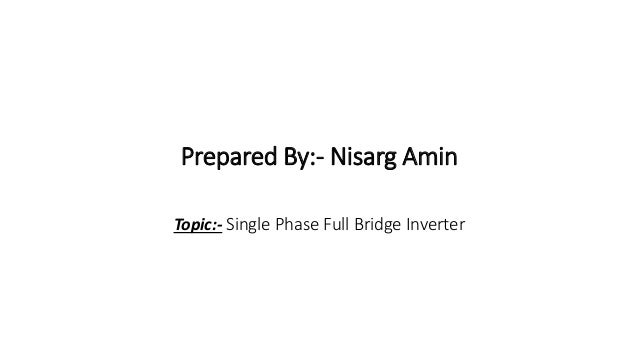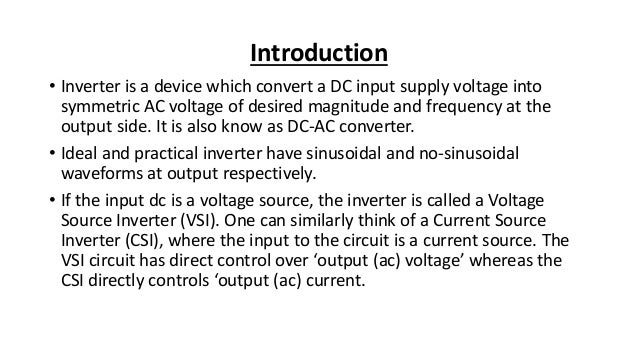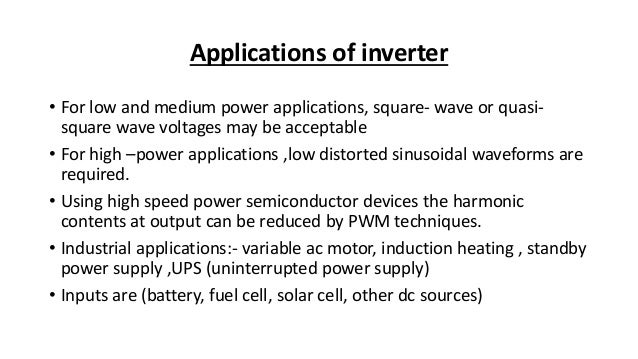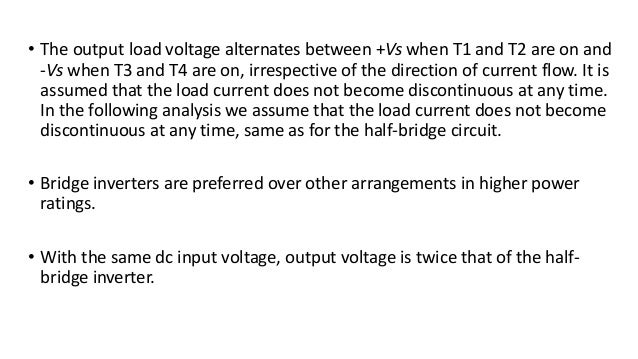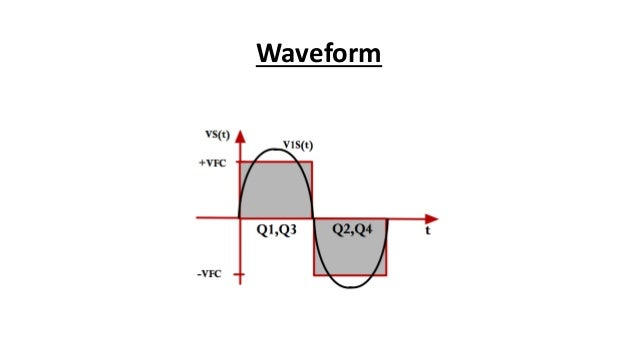Upcoming SlideShare
Next

23 Likes

Share

# Single phase full bridge inverter

Inverter is a device which convert a DC input supply voltage into symmetric AC voltage of desired magnitude and frequency at the output side. It is also know as DC-AC converter.
Ideal and practical inverter have sinusoidal and no-sinusoidal waveforms at output respectively.
If the input dc is a voltage source, the inverter is called a Voltage Source Inverter (VSI). One can similarly think of a Current Source Inverter (CSI), where the input to the circuit is a current source. The VSI circuit has direct control over ‘output (ac) voltage’ whereas the CSI directly controls ‘output (ac) current.

Inverter is a device which convert a DC input supply voltage into symmetric AC voltage of desired magnitude and frequency at the output side. It is also know as DC-AC converter.
Ideal and practical inverter have sinusoidal and no-sinusoidal waveforms at output respectively.
If the input dc is a voltage source, the inverter is called a Voltage Source Inverter (VSI). One can similarly think of a Current Source Inverter (CSI), where the input to the circuit is a current source. The VSI circuit has direct control over ‘output (ac) voltage’ whereas the CSI directly controls ‘output (ac) current.

See all

See all

### Single phase full bridge inverter

1. 1. Prepared By:- Nisarg Amin Topic:- Single Phase Full Bridge Inverter
2. 2. Introduction • Inverter is a device which convert a DC input supply voltage into symmetric AC voltage of desired magnitude and frequency at the output side. It is also know as DC-AC converter. • Ideal and practical inverter have sinusoidal and no-sinusoidal waveforms at output respectively. • If the input dc is a voltage source, the inverter is called a Voltage Source Inverter (VSI). One can similarly think of a Current Source Inverter (CSI), where the input to the circuit is a current source. The VSI circuit has direct control over ‘output (ac) voltage’ whereas the CSI directly controls ‘output (ac) current.
3. 3. Applications of inverter • For low and medium power applications, square- wave or quasi- square wave voltages may be acceptable • For high –power applications ,low distorted sinusoidal waveforms are required. • Using high speed power semiconductor devices the harmonic contents at output can be reduced by PWM techniques. • Industrial applications:- variable ac motor, induction heating , standby power supply ,UPS (uninterrupted power supply) • Inputs are (battery, fuel cell, solar cell, other dc sources)
4. 4. Classifications of inverter Inverter can be mainly classified into two types- a) Single-phase inverter b) Three-phase inverter Turn-ON and turn-OFF controlling devices are- a) Bipolar junction transistor[BJTs] b) Metal oxide semiconductor field-effect transistor[MOSFETs] c) Insulated-gate bipolar transistor[IGBTs] d) Gate turn-OFF thyristor[GTOs]
5. 5. Single Phase Full Bridge Inverter • Single phase full bridge inverter can be simplifying justified with a switching scheme of full bridge converter. • An square wave ac output voltage is synthesized from a dc input by closing and opening the switches in an appropriate sequence. • The output voltage can be +Vdc, -Vdc, or zero, depending on which switches are closed.
6. 6. Operation Of Single Phase Full Bridge Inverter • It consists of four choppers. Full bridge converter is also basic circuit to convert dc to ac. An ac output is synthesized from a dc input by closing and opening switches in an appropriate sequence. There are also four different states depending on which switches are closed. • When transistors T1 and T2 are turned on simultaneously, the input voltage appears across the load. If transistor and are turned on at the same time, the voltage across the is reversed and is –Vs. • Transistor T1 and T2 acts as switches S1 and S2. , respectively.
7. 7. Single Phase Full Bridge Inverter Equivalent Circuit
8. 8. When S1-S2 Turn ON & S3-S4 OFF For T1 < T < T2
9. 9. When S1-S2 Turn Off & S3-S4 On For T2 < T < T3
10. 10. • The output load voltage alternates between +Vs when T1 and T2 are on and -Vs when T3 and T4 are on, irrespective of the direction of current flow. It is assumed that the load current does not become discontinuous at any time. In the following analysis we assume that the load current does not become discontinuous at any time, same as for the half-bridge circuit. • Bridge inverters are preferred over other arrangements in higher power ratings. • With the same dc input voltage, output voltage is twice that of the half- bridge inverter.
11. 11. Waveform
12. 12. Analysis Of Full Bridge inverter • The fundamental RMS output voltage obtained from • RMS output voltage is • Fourier series of output voltage = 0 for n=2,4…. • For an RL load instantaneous load current i0 is            ....5,3,1 220 sin 4 n n S tn LnRn V i   S S rms V V V 90.0 2 4 )(01   SSrms VdVV      0 2 )(0 2            ,...5,3,1 0 sin 4 n S tn n V V  
•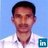#### DuraisamyP

Nov. 13, 2021
•Feb. 2, 2021
•Nov. 7, 2020
•#### vijay9944

Jun. 13, 2020
•#### sasikanthkola

May. 8, 2020
•#### TaufeeqSaheb

Mar. 26, 2020
•Feb. 16, 2020
•#### SaheliDey5

Dec. 10, 2019
•#### anushap1996

Nov. 13, 2019
•#### KumarSaurabh217

Apr. 14, 2019
•#### InduNagavarapu1

Feb. 9, 2019
•#### jaljoshi

Oct. 19, 2018
•#### DinesWaran2

Oct. 8, 2018
•May. 30, 2018
•#### TeenaShaju

May. 29, 2018
•#### SubhanAalam

May. 9, 2018
•#### SwarnashreeRoy

May. 5, 2018
•#### SahithAkula

Mar. 28, 2018
•#### JaiHunter1

Mar. 22, 2018
•#### jaiChoudhary8

Mar. 22, 2018

Total views

33,883

On Slideshare

0

From embeds

0

Number of embeds

11

589

Shares

0